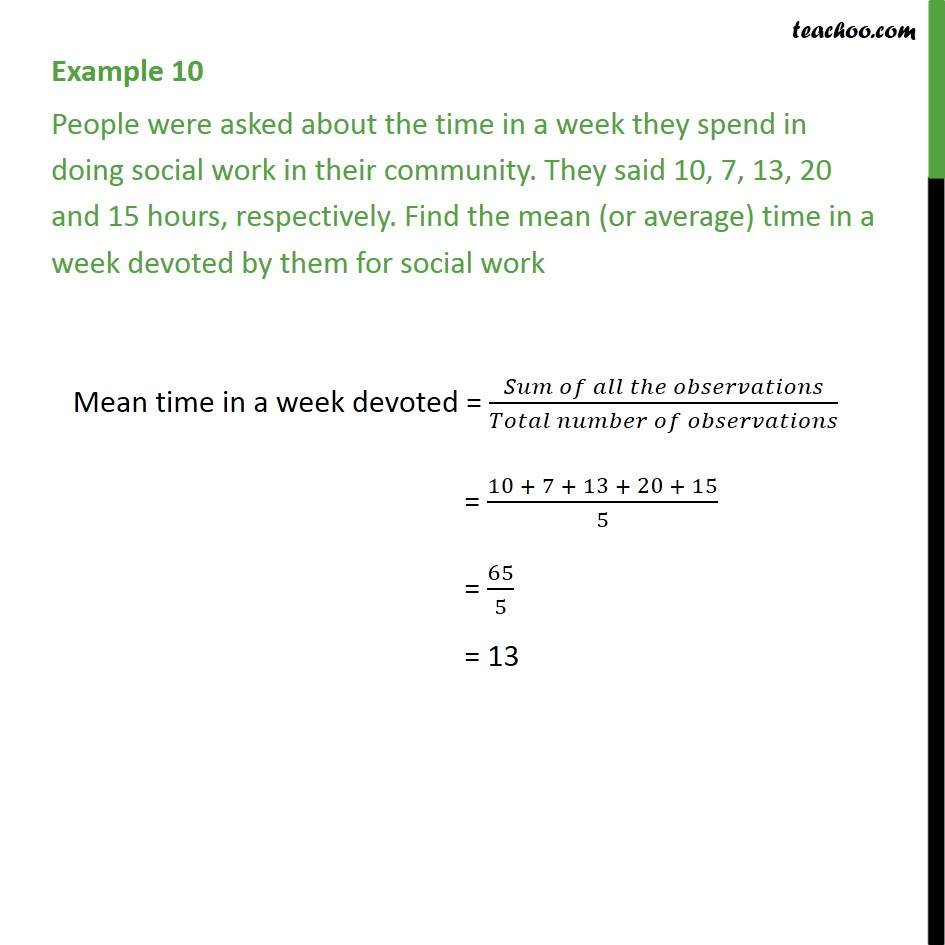1. Chapter 14 Class 9 Statistics (Term 1)
2. Serial order wise
3. Examples

Transcript

Example 10 People were asked about the time in a week they spend in doing social work in their community. They said 10, 7, 13, 20 and 15 hours, respectively. Find the mean (or average) time in a week devoted by them for social work Mean time in a week devoted = (𝑆𝑢𝑚 𝑜𝑓 𝑎𝑙𝑙 𝑡ℎ𝑒 𝑜𝑏𝑠𝑒𝑟𝑣𝑎𝑡𝑖𝑜𝑛𝑠)/(𝑇𝑜𝑡𝑎𝑙 𝑛𝑢𝑚𝑏𝑒𝑟 𝑜𝑓 𝑜𝑏𝑠𝑒𝑟𝑣𝑎𝑡𝑖𝑜𝑛𝑠) = (10 + 7 + 13 + 20 + 15)/5 = 65/5 = 13

Examples

Chapter 14 Class 9 Statistics (Term 1)
Serial order wise